# Analytical and numerical study of the dolichobrachistochrone problem

## Title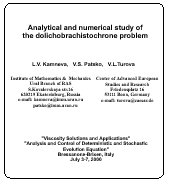This talk was presented at the conference "Viscosity Solutions and Applications" & "Analysis and Control of Deterministic and Stochastic Evolution Equation", Bressanone-Brixen, Italy, July 3-7, 2000. The paper is devoted to the analytical and numerical study of a time-optimal game problem in the plane. This problem is a game extension of the model brachistochrone problem.

## Slide 1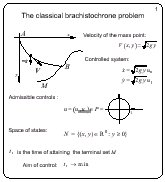Let A and B be arbitrary points in the vertical plane. One looks for a curve connecting A and B such that a mass point being started from A with zero initial velocity attains B along this curve for a minimal time. In general case, the terminal point B is replaced by a given terminal set M. For any trajectory, the velocity of the mass point at (x, y) is (2gy)1/2, where g is the gravitation constant.

The brachistochrone problem can be reformulated as a control problem: instead of choice of the trajectory, we assume that the magnitude of the velocity is (2gy)1/2 and the direction is defined by the unit vector u. Thus, u is chosen from the unit circle P. The problem is considered for y > = 0. The objective of the control u is to minimize the time of attaining a terminal set M. This control problem is equivalent to the brachistochrone problem.

## Slide 2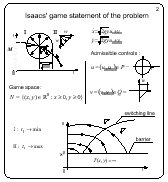When considering the brachistochrone problem in the book "Differential games", Isaacs introduced a disturbance influencing the dynamics of the system. The disturbance can be interpreted as a second player whose objective is to increase the time of attaining the terminal set.

Isaacs considered the first quadrant as the state space. The terminal set was the positive semiaxis y. So, it was unbounded. The vectograms of the players were: the circle of radius (2gy)1/2 for the first player and the diagonal of a square with the side w for the second player. The first player minimizes the time of attaining the terminal set M, the second player has the opposite objective.

It is clear that the coefficient (2g)1/2 does not play any role, hence it can be replaced by 1.

A solution to this differential game was given by Isaacs in his book. However, this solution is not fully correct. Namely, Isaacs supposed that the value function is infinite below the horizontal line y = w2. Above this horizontal line, the solution is defined by a switching line of the second player. On one side of the switching line, the second player utilizes one of two extremal controls. On the other side of the switching line, he uses the other extremal control.

## Slide 3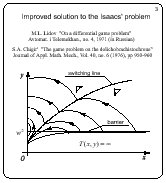Lidov pointed out to an error in the Isaacs' solution. Later on, Chigir has improved the solution of Isaacs. The horizontal line y = w2 is not a barrier, as it was erroneously claimed in the Isaacs' book. Namely, there are points below this line for which the game is solvable. The correct form of the barrier is shown on the slide. The optimal trajectories are breaking on the switching line of the second player. The value function is differentiable in the whole solvability domain.

It is interesting to modify the game statement of the brachistochrone problem so that the value function would become non-differentiable. Besides we wanted to find out whether singular equivocal lines can arise in the game brachistochrone problem. According to the book of Isaacs, equivocal singular lines are inherent to differential games only.

## Slide 4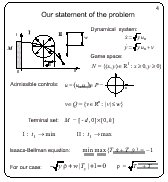Let the vectograms of the players are of the following form: a circle of radius y1/2 for the first player and a vertical segment of the length 2w for the second player. The symbols u1 and u2 denote control parameters of the first player in the description of the system dynamics. The variable v is a scalar control of the second player.

The terminal set M is chosen to be a rectangle of the height h with the base edge on the x-axis. Since the right-hand side of the dynamic equations does not depend on x, the location of M on the x-axis and its width do not play any essential role. This implies that the solution has to be symmetric with respect to the vertical line passing through the center of M.

A Bellman-Isaacs equation for this problem is written in the lower part of the slide.

Thus, we will consider only the right half of the solution. We begin with the case h > w2.

## Slide 5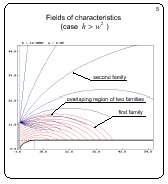Using the Isaacs' method, we obtain three families of characteristics that are constructed taking into account the boundary condition on the terminal set. The characteristics of the first, second, and third family emanate from the vertical part of the boundary, from the right upper vertex of M, and from the horizontal part of the boundary, respectively. The third family consists of vertical lines. For all families, the characteristics belonging to the same family do not intersect each other. The first and second families overlap partially. The second and third families adjoint each other smoothly.

Relations for the moving times along characteristics of all families are obtained. Unfortunately, they do not define the attainment time explicitly.

To obtain optimal trajectories, the characteristics of the first and second families were processed additionally.

## Slide 6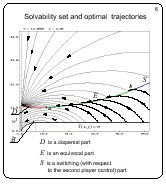The singular dispersal line D was computed from the condition that the attainment times along characteristics of the first and second families are equal. Parts of characteristics computed backwards are removed beyond intersection points with D. Each point of D is a source for two optimal trajectories. The construction of the dispersal line is stopped if it becomes tangent to one of the trajectories of the first family. A point corresponding to this situation is denoted by a on the slide.

The point a gives rise to a singular equivocal line E. The property of equivocal line is that two optimal trajectories emanate from every its point. One of these two optimal trajectories goes into the upper region and arrives at the right upper vertex of M along a characteristic of the second family. The second optimal trajectory goes along the equivocal line up to the point a.

The equivocal line is described by a first-order ordinary differential equation.

The construction of the equivocal line is continued until it meets a curve that bounds the second family from below. The equivocal line approaches this curve tangentially. Let us denote the common point by b. The point b divides the lower bounding curve of the second family into two parts: the right part is denoted by S on the slide. The curve S is a switching line of the second player.

On the second stage of solving process, characteristics of the Bellman-Isaacs equation are issued backwards from the parts E and S of the singular line. At the moment, complete description of these characteristics is absent (especially for the characteristics issued from the equivocal curve E).

Thus, the first arc of the singular line that defines the optimal solution possesses the dispersal property. This arc is computed numerically. The endpoint a of the dispersal arc can not be expressed analytically. The next arc of the singular line possesses the equivocal property. It is a numerical solution to a differential equation with a as the initial condition. The endpoint of the equivocal arc is determined numerically too. The third arc S is a switching line of the second player.

The value function is not differentiable on the arcs D and E, and it is differentiable on the line S. The solvability region of the problem is defined by the barrier line B that consists of a curvilinear segment and a horisontal line whose y-coordinate is equal to w2.

The barrier line is smooth. The value function is finite on the curvilinear part of the barrier. The second player can prevent the achievement of the terminal set whenever the trajectory starts from any point that lies below the barrier line.

It was the description of the solution structure for h > w2. Now, we show how the solution changes if the value of the parameter h decreases. The value of w is fixed.

## Slide 7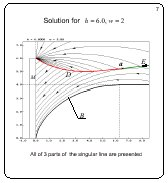For this slide, h = 6. For the previous slide, h = 12. Principally, the qualitative structure of the solution remains the same. As before, the singular line consists of three arcs and it is located over the barrier line B.

## Slide 8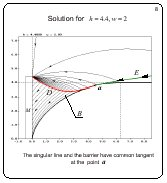If h continues to decrease, the singular line approaches the barrier line near and near. The limiting case where the singular line touches the barrier is shown.

## Slide 9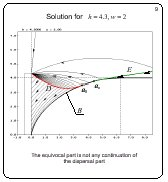If h decreases further, the singular line breaks up: the dispersal arc approaches the barrier B tangentially at the point a1 but the equivocal arc emanates tangentially from another point a2 lying on the barrier on the right from the point a1. Basically, the singular line consists of three parts as before.

## Slide 10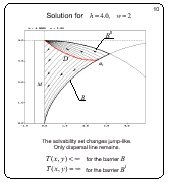If h = w2, the solvability region changes jump-like. An upper barrier line B* bounding the solvability region from above appears. The equivocal arc and switching arc of the second player disappear. It is interesting to observe that the value function is infinite on the upper barrier line B*. The value function is finite on the lower barrier B.

## Slide 11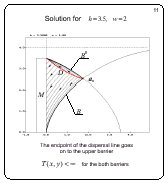If h decreases, the solution structure remains the same: two barriers define the solvability region. The endpoint of the dispersal arc goes on from the lower barrier to the upper barrier. The value function is finite for the both barriers.

These are results of our study based on the construction of the singular line. The solution is not trivial. We have a code for the computation of the singular line. The full exact proof for the described solution structure is not completed yet. Nevertheless, we are sure that our results are correct because they are in agreement with the independent computation of level sets of the value function. This independent computation utilizes an algorithm based on the backward propagation of fronts.

## Slide 12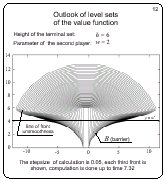Here, level sets of the value function are presented for h = 6, w = 2 . The curve consisting of the corner points of the fronts is clearly seen. This curve coincides with our singular line. The region that is filled out with the fronts coincides with the solvability region. The accumulation of the fronts near the horizontal line y = w2 means that the value function goes to infinity when approaching y = w2 from above.

## Slide 13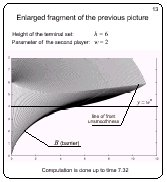Here, a fragment of the previous picture is shown. More fronts for the same time interval are presented.

## Slide 14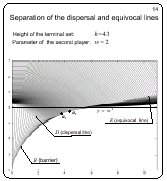On this slide, a fragment of the collection of level sets of the value function is shown for h = 4.3. One can see that the curve composed of corner points of the fronts is divided onto two parts. For h considered, the division points a1 and a2 are close to earch other.

## Slide 15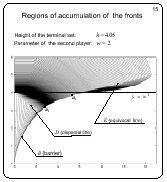If h decreases, the point a1 and a2 go away each from other. An accumulation of the fronts arise over the terminal set in the left part of the picture.

## Slide 16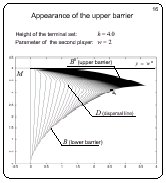This picture is done for h = w2 = 4. The accumulation of the fronts in the upper part of the picture gives the upper barrier B*. The solvability region changes jump-like.

## Slide 17If h decreases more, the upper accumulation region of the fronts reduces. The curve composed of the corner points of the fronts meets the lower barrier as before.

## Slide 18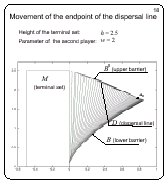The computation is done for h = 2.5. The curve composed of the corner points of the fronts meets the upper barrier.L.V. Kamneva, V.S. Patsko
Institute of Mathematics & Mechanics Ural Branch of RAS
S.Kovalevskaya str.16
620219 Ekaterinburg, Russia
e-mail:
kamneva@imm.uran.ru
patsko@imm.uran.ru

V.L.Turova
Center of Advanced European Studies and Research
Friedensplatz 16
53111 Bonn, Germany
e-mail:
turova@caesar.de

Patsko sector homepage